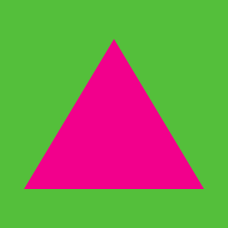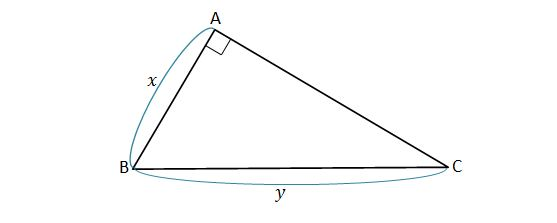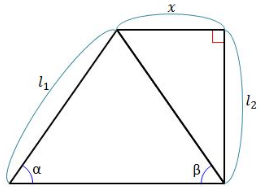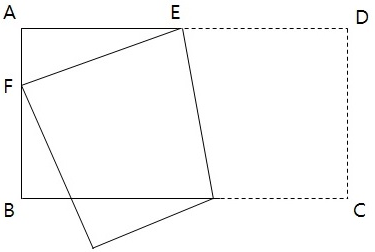Geometry

# Pythagorean TheoremIf $x=\sqrt{6}$ and $y=\sqrt{10}$ in right triangle $\triangle ABC$ shown in the diagram above, what is the length of $\overline{AC} ?$

Note: The above diagram is not drawn to scale.

Right triangle $ABC$ has side lengths $N+1, N-1,$ and $\frac {N}{2}.$

What is the value of $N?$In the figure above, if $l_1=10,$ $l_2=8$ and $\alpha=\beta={56}^\circ,$ what is the value of $x ?$

Note: The above diagram is not drawn to scale.As shown in the above diagram, Raj folded a rectangular paper $ABCD$ with side length $\overline{AD} = 5$ in such a way that vertex $D$ falls on side $AB$ and is labeled point $F.$ If the length of $\overline{AF}$ is $2,$ what is the length of $\overline{DE}?$

Note: The above diagram is not drawn to scale.

Dan walks 30 meters east. He then walks 40 meters north. How far (in meters) is he from the starting point?

×